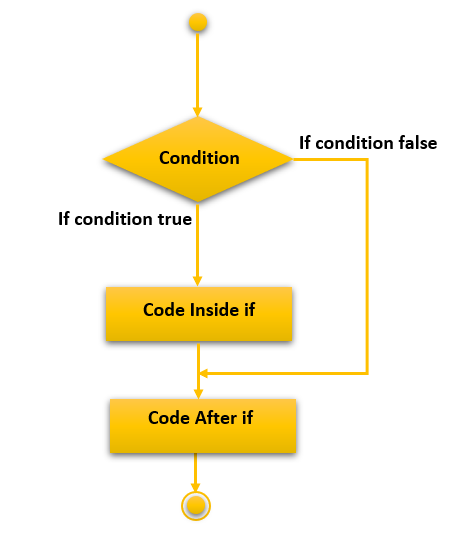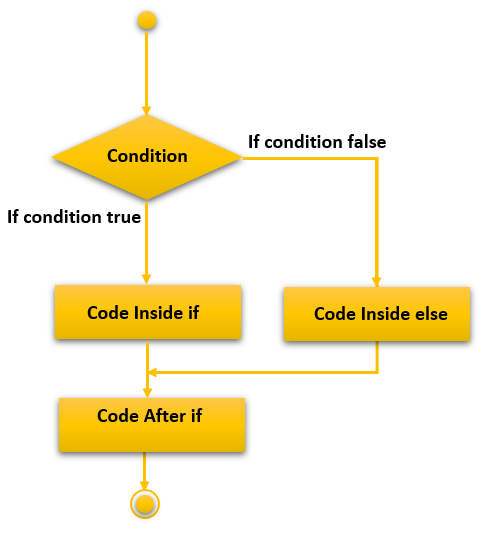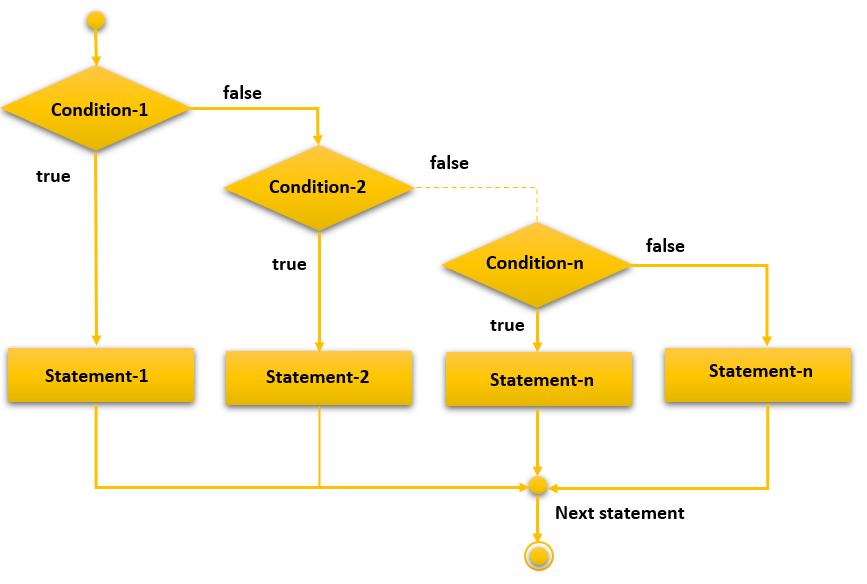# C if else Statement

In this tutorial, you will learn about the if statement including if…else, if…else…if ladder, and nested if…else in C programming language with the help of examples.

The if..else statement in the C programming language is used as a control statement. It performs operations based on some specific condition. The group of statements used to execute only if the specified condition is true.

There are the following variants of the if statements available in C language.

• If statement
• If-else statement
• Nested if

## C if Statement

The if statement is used to perform certain operations only if a given condition is true.

### Syntax

The syntax of the if statement is given below.

```if(test expression){
//code to be executed
}```

### Flowchart of if statement in C### Example

Let’s check a simple example of `if` statement of C language.

```// Program to display a message if it is less than 50

#include <stdio.h>
int main() {
int i;

printf("Enter an integer: ");
scanf("%d", &i);

// true if number is less than 50
if (i < 50) {
printf("The number is less than 50.\n");
}

printf("You entered %d.", i);
return 0;
}```

### Output 1

Enter an integer: 35
The number is less than 50.
You entered 35.

We enter the number `35`. Hence the body the `if` condition executed and the message `The number is less than 50.` gets printed.

### Output 2

Enter an integer: 75
You entered 75.

Now, we have entered a number that is greater than `50`. So the body of the `if` condition not executed.

### Program to find if the given number is an even number.

```// Program to check if the given number is even

#include<stdio.h>
int main(){

int i=0;
printf("Enter a number:");
scanf("%d",&i);

if(i%2==0){
printf("%d is even number",i);
}

return 0;
}```

### Output

Enter a number:14
14 is an even numberEnter a number:15

## C if…else Statement

The if…else statement is the extension of the if statement. The if statement performs an operation only if the given condition is correct. Using the if…else statement two operations can be performed i.e. one for the correctness of the given condition another is incorrectness of the given condition. Here, you must remember that the if and else block cannot be executed simultaneously. Either of the ones will be executed.

### Syntax

The syntax of the if…else statement is as follows.

```if(test expression){
//code to be executed if condition is true
}else{
//code to be executed if condition is false
}```

### Flowchart of if…else statement in C### Example

Let’s check a simple example of the if…else statement in C language.

```// Example of if...else statement in C

#include <stdio.h>
int main() {
int i;

printf("Enter an integer: ");
scanf("%d", &i);
printf("You entered %d.\n", i);

// true if number is less than 50
if (i < 50) {
printf("The number is less than 50.\n");
}

// execute if the number is greater than 50
else {
printf("The number is greater than 50.");
}
return 0;
}```

### Output 1

Enter an integer: 35
You entered 35.
The number is less than 50.

Here, the number is `35` which is less than `50`. Hence, the `if` block gets executed.

### Output 2

Enter an integer: 75
You entered 75.
The number is greater than 50.

Here, the number is `75` which is greater than `50`. Hence, the `else` block gets executed.

The if…else statement checks for two possibilities depending upon whether the test expression is true or false. Sometimes it is required to make decisions from more than two choices.

The if…else allows you to check more than two possibilities and perform different operations according to the test conditions.

### Syntax

The syntax of if…else ladder in C language as follows.

```if (test expression1) {
// code to be executed if test expression1 is true
}
else if(test expression2) {
// code to be executed if test expression2 is true
}
else if (test expression3) {
// code to be executed if test expression3 is true
}
.
.
else {
// code to be executed if all the test expressions are false
}```

### Flowchart of if…else ladder statement in C### Example

```// Example of the if...else ladder in C

#include <stdio.h>
int main() {
int i;

printf("Enter a positive integer: ");
scanf("%d", &i);
printf("You entered %d.\n", i);

// true if number is less than 50
if (i < 50) {
printf("The number is less than 50.\n");
}
// execute if the number is greater than 50 and less than 100
else if(i>50 && i<100){
printf("The number is grater than 50 and less than 100.\n");
}
// execute if the number is greater than 100
else {
printf("The number is greater than 100.");
}
return 0;
}```

### Output

Enter a positive integer: 40
You entered 40.
The number is less than 50.Enter a positive integer: 80
You entered 80.
The number is greater than 50 and less than 100.Enter a positive integer: 120
You entered 120.
The number is greater than 100.

## C Nested if…else

It is also possible to place an if…else statement inside the body of another if..else statement. Note that the same thing also can be achieved using the if…else ladder.

### Example

```// Example of nested if..else statement in C

#include <stdio.h>
int main() {
int i;

printf("Enter an integer: ");
scanf("%d", &i);
printf("You entered %d.\n", i);

// true if number is less than 50
if (i < 50) {
if(i<20){
printf("The number is less than 20.\n");
}
else {
printf("The number is less than 50.\n");
}
}

// execute if the number is greater than 50
else {
printf("The number is greater than 50.");
}
return 0;
}```

### Output

Enter an integer: 15
You entered 15.
The number is less than 20.Enter an integer: 30
You entered 30.
The number is less than 50.Enter an integer: 60
You entered 60.
The number is greater than 50.